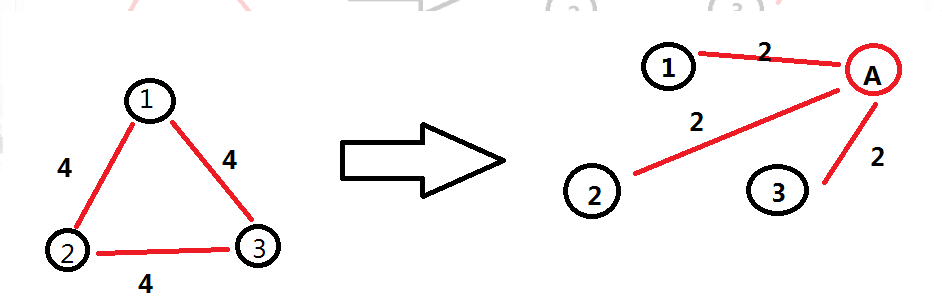# Meeting

Time Limit: 12000/6000 MS (Java/Others)    Memory Limit: 262144/262144 K (Java/Others)
Total Submission(s): 5241    Accepted Submission(s): 1647

Problem Description

Bessie and her friend Elsie decide to have a meeting. However, after Farmer John decorated his
fences they were separated into different blocks. John's farm are divided into n blocks labelled from 1 to n.
Bessie lives in the first block while Elsie lives in the n-th one. They have a map of the farm
which shows that it takes they ti minutes to travel from a block in Ei to another block
in Ei where Ei (1≤im) is a set of blocks. They want to know how soon they can meet each other
and which block should be chosen to have the meeting.

Input

The first line contains an integer T (1≤T≤6), the number of test cases. Then T test cases
follow.

The first line of input contains n and m. 2≤n≤105. The following m lines describe the sets Ei (1≤im). Each line will contain two integers ti(1≤ti≤109) and Si (Si>0) firstly. Then Si integer follows which are the labels of blocks in Ei. It is guaranteed that ∑mi=1Si≤106.

Output

For each test case, if they cannot have the meeting, then output "Evil John" (without quotes) in one line.

Otherwise, output two lines. The first line contains an integer, the time it takes for they to meet.
The second line contains the numbers of blocks where they meet. If there are multiple
optional blocks, output all of them in ascending order.

Sample Input

2 5 4 1 3 1 2 3 2 2 3 4 10 2 1 5 3 3 3 4 5 3 1 1 2 1 2

Sample Output

Case #1: 3 3 4 Case #2: Evil John

Hint

In the first case, it will take Bessie 1 minute travelling to the 3rd block, and it will take Elsie 3 minutes travelling to the 3rd block. It will take Bessie 3 minutes travelling to the 4th block, and it will take Elsie 3 minutes travelling to the 4th block. In the second case, it is impossible for them to meet.

Source

2015ACM/ICPC亚洲区沈阳站-重现赛（感谢东北大学）#include <bits/stdc++.h>
typedef long long ll;
const int MAXM = 3000006;
const int MAXN = 200005 + MAXM;
const ll INF = 1e18;
using namespace std;
struct Edge
{
int to,Next;
ll w;
}edge[MAXN];
int tot,head[MAXN],n,m;
void init()
{
tot = 0;
memset(head,-1,sizeof(head));
}
void addedge(int u,int v,ll w)
{
edge[tot].to = v;
edge[tot].w = w;
edge[tot].Next = head[u];
head[u] = tot++;
}
struct node
{
int u;
ll dis;
node(){}
node(int _u,ll _dis)
{
u = _u;
dis = _dis;
}
bool operator < (const struct node& a) const
{
return dis > a.dis;
}
};
ll dis1[MAXN],dis2[MAXN];
bool vis[MAXN];
void Dijsktra(int s,ll dis[])
{
struct node t;
int u,v;
for(int i = 1; i <= n + m; i++) {
dis[i] = INF;
vis[i] = false;
}
priority_queue<node> pq;
dis[s] = 0;
pq.push(node(s,dis[s]));
while(!pq.empty()) {
t = pq.top();
pq.pop();
u = t.u;
if(vis[u]) continue;
vis[u] = true;
for(int i = head[u]; i != -1; i = edge[i].Next) {
v = edge[i].to;
if(dis[v] > dis[u] + edge[i].w) {
dis[v] = dis[u] + edge[i].w;
pq.push(node(v,dis[v]));
}
}
}
}
vector<int> vc;
int main(void)
{
int T,num;
int u,v;
ll w,ans;
int kase = 0;
scanf("%d",&T);
while(T--) {
kase++;
init();
scanf("%d %d",&n,&m);
v = n;
for(int i = 1; i <= m; i++) {
scanf("%I64d %d",&w,&num);
v++;
for(int j = 1; j <= num; j++) {
scanf("%d",&u);
addedge(u,v,w);
addedge(v,u,w);
}
}
Dijsktra(1,dis1);
Dijsktra(n,dis2);
ans = INF;
for(int i = 1; i <= n; i++) {
ans = min(ans,max(dis1[i],dis2[i]));
}
vc.clear();
for(int i = 1; i <= n; i++) {
if(max(dis1[i],dis2[i]) == ans) {
vc.push_back(i);
}
}
if(ans == INF) {
printf("Case #%d: Evil John\n",kase);
}
else {
printf("Case #%d: %d\n",kase,ans / 2);
for(int i = 0; i < vc.size(); i++) {
if(i == 0) printf("%d",vc[i]);
else printf(" %d",vc[i]);
}
printf("\n");
}
}
return 0;
}